American Journal of Theoretical and Applied Statistics
Volume 4, Issue 6, November 2015, Pages: 640-643

Methodology Article

Detection of Multicollinearity Using Min-Max and Point-Coordinates Approach

Umeh Edith Uzoma1, Awopeju Kabir Abidemi1, Ajibade F. Bright2

1Department of Statistics, Nnamdi Azikiwe University, Awka, Anambra State, Nigeria

2Department of General Studies, Petroleum Training Institute, Effurun, Delta State, Nigeria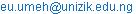(U. E. Uzoma)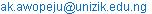(A. K. Abidemi)(A. F. Bright)

Umeh Edith Uzoma, Awopeju Kabir Abidemi, Ajibade F. Bright. Detection of Multicollinearity Using Min-Max and Point-Coordinates Approach. American Journal of Theoretical and Applied Statistics. Vol. 4, No. 6, 2015, pp. 640-643. doi: 10.11648/j.ajtas.20150406.36

Abstract: Multicollinearity is one of the problems or challenges of modeling or multiple regression usually encountered by Economists and Statisticians. It is a situation where by some of the independent variables in the formulated model are significantly or highly related/correlated. In the past, methods such as Variance Inflation Factor, Eigenvalue and Product moment correlation have been used by researchers to detect multicollinearity in models such as financial models, fluctuation of market price model, determination of factors responsible for survival of man and market model, etc. The shortfalls of these methods include rigorous computation which discourages researchers from testing for multicollinearity, even when necessary. This paper presents moderate and easy algorithm of the detection of multicollinearity among variables no matter their numbers. Using Min-Max approach with the principle of parallelism of coordinates, we are able to present an algorithm for the detection of multicollinearity with appropriate illustrative examples.

Keywords: Variance Inflation Factor, Matrix, Eigen Values, Characteristics Root, Range, Gradient

Contents

1. Introduction

Modeling usually involves more than one independent variable and in many cases, researchers find it difficult to select most significant variables among all. The process is called Parsimony in Econometrics. In other field of endevour, due to inadequate knowledge of Modeling, some researchers neglect harmful effect of multicollinearity in the model formulated. According to literature, this may result to inappropriate model, erroneous conclusion and sometimes insignificant parameters with significant model (Hawking and Pendleton, 1983; Kennedy, 2002; Gujrati, 2004; Vaughan and Berry, 2005). According to Belsley (1991), multicollinearity can lead to large changes in a model, even, resulting to changes of sign of parameter estimates. The best regression models are those in which the independent variables each correlate highly with the dependent (outcome) variable but correlate at most only minimally with each other, such model is often called "lownoise" and statistically robust (Kock and Lynn, 2012). Multicollinearity is commonly encountered by researchers when dealing with modeling which involves more than one independent variable. By definition, it is a situation where independent variables in a model are highly and significantly related (Wetheriletal, 1986; Draper and Smith, 2003). In the past and recent time, a lot of methods exist for the detection of multicollinearity and many researchers suggested ways of curbing the menace which include remodeling and parsimony. See Montgometry, et al (2001).

According to Jim (2013), moderate multicollinearity may not be problematic but severe multicollinearity is a problem because it can increase the variance of the coefficient estimates and make estimates sensitive to changes in the model, thereby, causing instability in the coefficient estimates which may be difficult to interpret. The researcher further stated that multicollinearity saps statistical power of the analysis, can cause the coefficients to switch signs, and makes it more difficult to specify the correct model.

According to Investopedia’s report on multicollinearity, multicollinearity suggests that several of the independent variables are closely linked. Once the collinear variables are identified, it may be helpful to study whether there is a causal link between the variables. One of the ways of resolve multicollinearity problems is to reduce the number of collinear variables until there is only one remaining out of the set. Sometimes, after some studies, it may be possible to identify one of the variables as being extraneous. Alternatively, it may be possible to combine two or more closely related variables into a single input.

Statistical issue with multicollinearity is fairly simple. The unique effects of individual predictors are estimated by holding all other predictors constant and thus ignoring any shared variance between predictors. A regression model uses information about the variation between predictors and the associated variation in the outcome (y variable) to calculate estimates. As n (the number of participants or cases or sample size) increases, the more information and the greater the statistical power of the analysis. If multicollinearity is present, then, each data point tends to contribute less information to the estimate of individual effects than it does to the overall analysis.

Methods used by researchers for the detection of multicollinearity in modeling include;

1. Product moment Correlation.

2. EigenValues.

3. Variance Inflation Factor.

All the methods have proven to be suitable and have been used for a very long time, especially, in applications. Considering the steps involve in the computation using the methods, researchers with phobia for lengthy calculation or computation often find it difficult to compute the process most especially, Eigenvalues which involves matrix operation and Variance Inflation Factor (VIF) which involves computation of coefficient of determination for all possible regression (Brien, 2007). Product moment correlation involves computation of correlation between variables and test of significance of the correlation value (Belsley, 1991; Kumar, 1975).

Economists and Policymakers often use regression for modeling and are much familiar with Product Moment Correlation or Variance Inflation Factor for the detection of multicollinearity. Some as a result of ignorance, copy verbatim the output of software without investigation or test of multicollinearity. Close contact with some of the researchers reveals it was deliberate action to prevent tedious computation or to boycott mathematical computation. This calls for more friendly approach of the detection of multicollinearity in modeling to prevent reoccurrence of such act and prevent future occurrence of such action due to transfer of knowledge by the trainers who are not well equipped in the field of modeling or statistical computing.

In this paper, a new approach to the computation or detection of multicollinearity is presented with 5-steps algorithm which is user friendly and requires less mathematical rigor.

2. Propose Method

The method is called "Min-Max". Min-Max involves computation of gradient of line drawn using the points/observations of the variables of interest. In the principle of coordinates, two lines are said to be parallel if their gradient is equal and perpendicular if the product of their gradients is negative 1 (MathCentre, 2009).

Using the principle stated above, two significantly related independent variables can only produce two unparallel lines which if extended meet at the long run, otherwise, the variables are not significantly related which can be interpreted as absence of multicollinearity.

Necessary Assumption

Two lines are said to be parallel if they have common gradient and perpendicular if the product of their gradients is equal to -1[Mathcentre, 2009].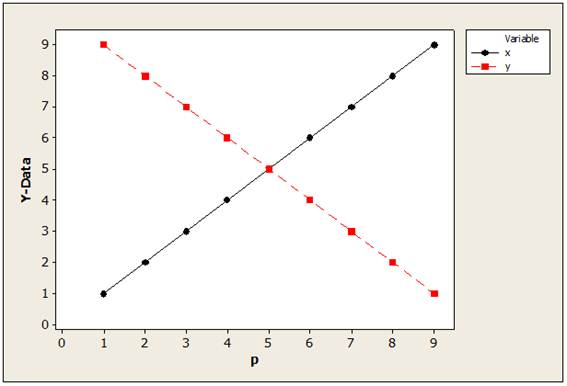Figure 1. Sample of unparallellines.

As shown in figure1, the lines are not parallel as they meet at a point and one should not expect their gradient to be equal. Then, how can multicollinearity be detected using the parallelism principle?

Min-Max: using maximum point and minimum points in the independent variables of interest.

Point-coordinates: this is used to detect parallelism of lines formulated as a result of min-max which eventually serves as point coordinates.

Algorithm.

Rearrange the observations in ascending order.

Attach serial numbers to the observations.

Locate minimum and maximum observations for variables.

Plot scatter diagram of least serial and maximum serial numbers against min-max of variables.

Proof that the lines are parallel using coordinates of points.

Note that Gradient is equivalent to ratio of range of variables if minimum and maximum points are used in the computation of gradient. That is,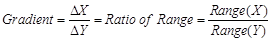(1)

If change in Y and change in X are computed using Min-Max.

3. Illustrative Example

Using the data below, show whether the variables are significantly related with the aid of Min-Max.

Table 1. Observed Data.

 S/N 1 2 3 4 X1 1 3 4 5 X2 2 6 8 10

Solution:

Table 2. Observed data with ranks.

 S/N X1 X2 Rx1 Rx2 1 1 2 1 2 2 3 6 3 6 3 4 8 4 8 4 5 10 5 10

Let S/N be the independent variable and Rx1 and Rx2 be the dependent variables.

Using Min-Max, coordinates of Rx2 is (4, 10) and (2, 1). Likewise, Rx1 is (5, 4) and (1, 1).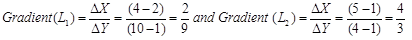L1 not same as L2 which implies the lines drawn from the observations are not parallel and thus, the variables are significantly related. Therefore, multicollinearity exists.

Diagrammatically.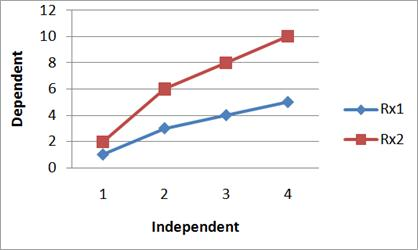Figure 2. Scatter Plot of the observations from the two variables.

The figure shows that the lines drawn from the coordinates are not parallel to each other which implies the lines could meet if extended beyond the present length. It is an indication that the variables are significantly related and should not be combined in modeling or multiple regression. The figure supports the conclusion from Min-Max computation using gradient of the coordinates.

Sample two.

Table 3. Observed Data.

 S/N A B X 1 1 9 2 2 2 8 4 3 3 7 6 4 4 6 8 5 5 5 10 6 6 4 12 7 7 3 14 8 8 2 16 9 9 1 18

The data/observations are ordered which requires no ranking as shown in the previous example. The independent variable is ‘S/N’ and dependent variables are ‘A’, ‘B’ and ‘X’. (see Table 2). Min-Max of ‘A’ are (1, 1) and (9, 9); ‘B’ are (9, 1) and (1, 9); ‘X’ are (2, 1) and (18, 9). Gradients are: 0, -1 and 2 respectively.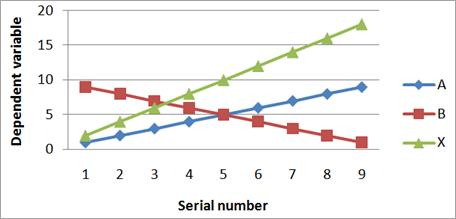Figure 3. Scatter plot of the three variables.

Point of coordinates can be detected from the lines drawn from observations, extreme values are considered to get perfect result. As shown in the figure 3, more variables can be accommodated which qualifies the method for multivariate approach. The figure shows that lines produced by the observations of the variables are not parallel therefore, they are related and that can be tagged multicollinearity among independent variables. Applying existing method; Product Moment Correlation, for the data on Table 3, we now obtained;

Table 4. Correlation Matrix.

 A B X A 1 B -1 1 X 1 -1 1

Considering proposed method with existing method, Product Moment Correlation was used as it is one of the existing methods for the detection of multicollinearity among independent variables. As shown in Table 4, Product Moment Correlation shows that the variables are significantly related which proves the existence of multicollinearity among the variables. This is in line with the conclusion of the proposed method but the proposed method is better as it is easy to compute with diagrammatic illustration.

4. Summary of Findings

Multicollinearity is a problem in modeling which can render a model formulated useless and if care is not taken, researchers may not know that the model is faulty. Ignoring the rigor of lengthy computation, Min-Max as presented can be used to detect multicollinearity. The algorithm can be used for both univariate and multivariate with fewer rigors. The propose method can be used by even non-mathematicians as it requires less/ non-rigorous computation. This made it superior to other existing methods.

Illustrative examples were used to show how to use the algorithm and to display its simplicity for even three independent variables. The illustrative examples show that the technique is appropriate for the detection of multicollinearity.

It is widely acceptable to use any point at the line of observations for the computation of gradient especially when dealing with straight line. Therefore, the concept of Min-Max is mathematically accurate in the computation of gradient points. Also, based on the statement on parallel line, it is adequate to use position of lines for conclusion whether two or more variables are related or not (linearly related).

In another context, the algorithm can be used by researchers in other fields of endevour for the determination of fixed points of locations. It shows the link between range and gradient when minimum and maximum points are used. Therefore, it is not only good for detection of multicollinearity but also fix-point determination.

Future study can still be done on the test statistic thereby modified for easier and shorter algorithm other than the approach used in this paper. Simulated study can be adopted in the validation of test statistic other than what researchers used.

References

1. Brien, R. M. (2007). "A Caution Regarding Rules of Thumb for Variance Inflation Factors". Quality & Quantity 41(5): 673.doi:10.1007/s11135-006-9018-6.
2. Belsley, D. (1991). Conditioning Diagnostics: Collinearity and Weak Data in Regression. New York: Wiley.ISBN0-471-52889-7.
3. Draper, N. R., Smith, H. (2003). Applied regression analysis, 3rd edition, Wiley, New York.
4. Gujrati, D. N. (2004). Basic econometrics 4th edition, Tata McGraw-Hill, New Delhi.
5. Hawking, R. R. and Pendleton, O. J. (1983). "The regression dilemma", Commun. Stat.-Theo. Meth, 12,497-527.
6. Jim F. (2013). "What Are the Effects of Multicollinearity and When Can Ignore Them?"http://blog.minitab.com/blog/adventures-in-statistics/what-are-the-effects-of-multicollinearity-and-when-can-i-ignore-them.Assessed:17th,Dec.,2015.
7. Johnston, J. and Dinardo, J. (1997) Econometric methods, 4th edition, McGraw-Hill, Singapore.
8. Kennedy, P. E. (2002), "More on Venn Diagrams for Regression," Journal of Statistics Education [Online], 10(1). (www.amstat.org/publications/jse/v10n1/kennedy.html).
9. Kumar, T. K. (1975). "Multicollinearity in Regression Analysis".Review of Economics and Statistics57(3): 365–366.JSTOR1923925.
10. Kock, N.; Lynn, G. S. (2012)."Lateral collinearity and misleading results invariance-based SEM: An illustration and recommendations".Journal of the Association for Information Systems13(7): 546–580.
11. MathCentre (2009); Equations of Straight Lines.www.mathcentre.ac.uk/resources/uploaded/mc-ty-strtlines-2009-1.pdf. Assessed: 10/12/2015.
12. Montgomery, D. C., Peck, E. A., Vining, G. G. (2001). Introduction to linear regression analysis, 3rd edition, Wiley, NewYork.
13. Vaughan, T. S., and Berry, K. E. (2005): "Using Monte Carlo Techniques to Demonstrate the Meaning and Implications of Multicollinearity". Journal of Statistics Education. Vol.13, Number 1.
14. Wetherill, G. B., Duncombe, P., Kenward, M., Kollerstrom,J. (1986). Regression analysis with application, 1st edition, Chapman and Hall, New York.

 Contents 1. 2. 3. 4.
Article ToolsAbstractPDF(405K)